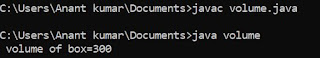## Wednesday, 1 August 2018

### Program to print n terms of fibonacci series in c

/* program to print fibo series */
#include <stdio.h>
void main ()
{
int a=0,b=1,sum,counter=0,n ;
clrscr () ;
printf ("/n %d",a) ;
printf ("/n %d",b) ;
printf ("/n how many terms you want to print:") ;
scanf ("%d", & n) ;
while (counter<n-2)
{
sum=a+b ;
printf ("/n %d",sum) ;
a=b ;
b=sum ;
counter++ ;
}
getch () ;
}

### Output :

How many terms you want to print : 5
number are : 0 , 1 , 1 , 2 , 3.

### Algorithm ;;

ALGORITHM : FIBONACCI SERIES ( A , B , N , COUNTER )

This algorithm is used to print n terms of fibonacci series

STEP 1. START.
STEP 2. SET A = 0 , B = 1 , COUNTER = 0 .
STEP 3. INPUT N.
STEP 4. WRITE A.
STEP 5. WRITE B.
STEP 6. REPEAT STEP 7 TO 11.
COUNTER < N -2
[ N - 2 IS WRITTEN BECAUSE OF PRINTING FIRST TWO TERMS OUT OF LOOP ]
STEP 7. SUM : = A + B.
STEP 8. PRINT SUM.
STEP 9. SET A : = B.
STEP 10. SET B : = SUM.
STEP 11. [ INCREMENT THE VALUE OF COUNTER BY 1 ]
COUNTER : = COUNTER + 1.
STEP 12. STOP.